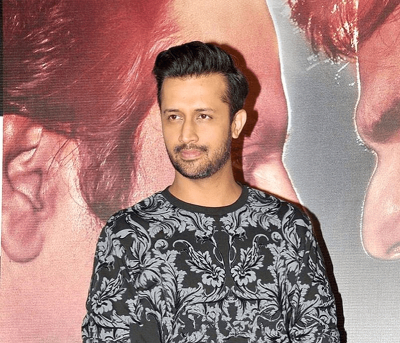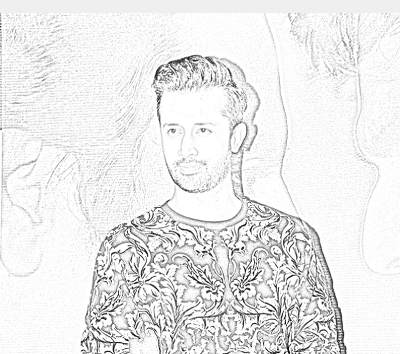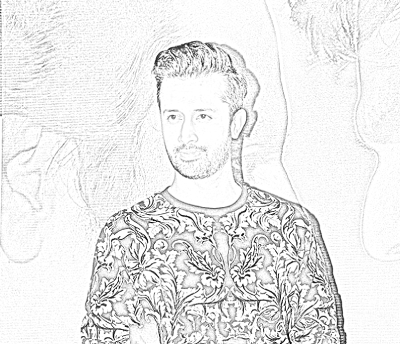• +91-9872993883
• +91-8283824812
• info@ris-ai.com

# Pencil Sketch of a photo ¶

### Overview of pencil sketch on a photo ¶

we disscuss all the steps regarding the project one by one below:

1. First, we take an image that we want to convert to a pencil sketch. I am using the image of a singer name Atif aslam.
2. Next, we need to read the image in RBG format and then convert it to a grayscale image. This will turn an image into a classic black and white photo.
3. Then the next thing to do is invert the grayscale image also called negative image, this will be our inverted grayscale image. Inversion can be used to enhance details.
4. Then we can finally create the pencil sketch by mixing the grayscale image with the inverted blurry image. This can be done by dividing the grayscale image by the inverted blurry image.

Since images are just arrays, we can easily do this programmatically using the divide function from the cv2 library in Python.

### OpenCV library¶

The only library we need for converting an image into a Pencil Sketch with Python is OpenCV library in Python.

In :
import cv2


In [ ]:
image = cv2.imread("/Atif2.jpg")
cv2.imshow("orijinal-image", inverted_image)
cv2.waitKey(0)### converting the original image to greyscale:¶

In [ ]:
gray_image = cv2.cvtColor(image, cv2.COLOR_BGR2GRAY)
cv2.imshow("New Dog", gray_image)
cv2.waitKey(0)### invert the new grayscale image: ¶

In [ ]:
inverted_image = 255 - gray_image
cv2.imshow("Inverted", inverted_image)
cv2.waitKey()### Using the Gaussian Function in OpenCV ¶

In [ ]:
blurred = cv2.GaussianBlur(inverted_image, (21, 21), 0)

In [ ]:
inverted_blurred = 255 - blurred
pencil_sketch = cv2.divide(gray_image, inverted_blurred, scale=256.0)
cv2.imshow("Sketch", pencil_sketch)
cv2.waitKey(0)In [ ]:


In [ ]: